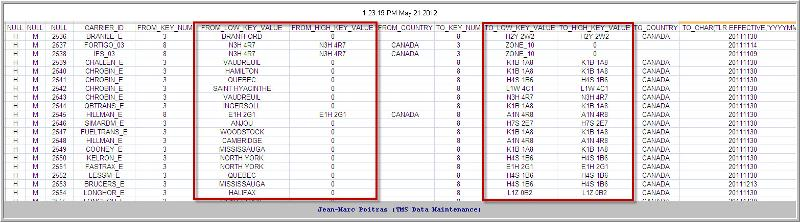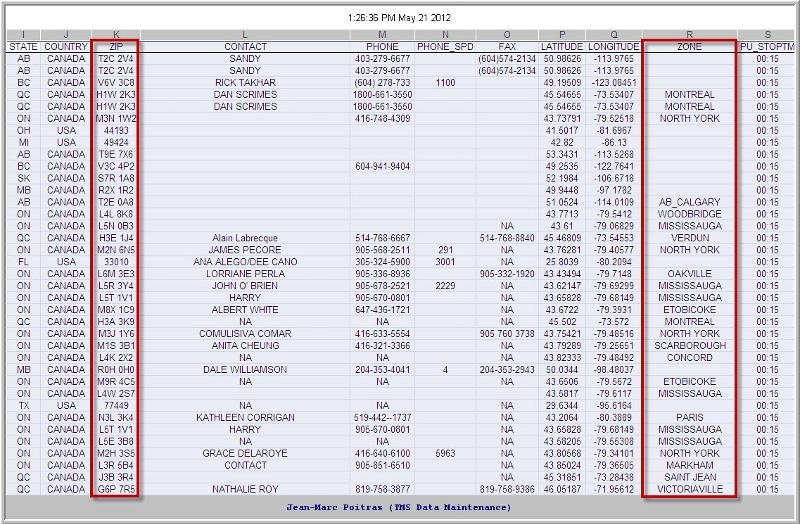# SQL to search zone and zipon
Hello All

I have 2 SQL, 1 that pull all rates:
``````SELECT
TLR.CARRIER_ID,
LRN.FROM_KEY_NUM,
LRN.FROM_LOW_KEY_VALUE,
LRN.FROM_HIGH_KEY_VALUE,
LRN.FROM_COUNTRY,
LRN.TO_KEY_NUM,
LRN.TO_LOW_KEY_VALUE,
LRN.TO_HIGH_KEY_VALUE,
LRN.TO_COUNTRY
from TL_RATE tlr, TL_RATE_DETAIL trd, LANE_RATE_NETWORK lrn
WHERE tlr.CARRIER_ID = trd.CARRIER_ID (+)
and tlr.LANE_ID = trd.LANE_ID (+)
and tlr.TARIFF_CLASS_ID = trd.TARIFF_CLASS_ID (+)
and tlr.EFFECTIVE = trd.EFFECTIVE (+)
and tlr.LANE_ID = lrn.ID
and tlr.EXPIRATION > to_date(to_char(sysdate,'YYYYMMDD'), 'YYYYMMDD');
``````

And one that will Pull all locations:
``````SELECT
ID,
LOC_TYPE_ID,
NAME,
CITY,
STATE,
COUNTRY,
ZIP,
CONTACT,
PHONE,
PHONE_SPD,
FAX,
LATITUDE,
LONGITUDE,
ZONE
from location
``````

Now what i would like to do is this:

I would like to validate if i have any ZONE or ZIP that are wrong in the rate table, if i validate it with all the ZONES and ZIP from my location table.

At the end, i just want a result with the rates with bad ZIP and ZONE.

Now to determine the zip and the zone from the rate table is like this:

If LRN.FROM_KEY_NUM = 3, then that equals to ZONE.
If LRN.FROM_KEY_NUM = 8, then that equals to ZIP.

Same for LRN.TO_KEY_NUM:
If LRN.TO_KEY_NUM = 3, then that equals to ZONE.
If LRN.TO_KEY_NUM= 8, then that equals to ZIP.

The ZONE or the ZIP from the rate table will be in column:
``````LRN.FROM_LOW_KEY_VALUE,
LRN.FROM_HIGH_KEY_VALUE,
LRN.TO_LOW_KEY_VALUE,
LRN.TO_HIGH_KEY_VALUE,
``````

and in the Location table, the zone or the zip will be in columns:
``````ZIP,
ZONE
``````

How can i extract all zip and zone that do not exist in the location tab?

Thanks
Comment
Watch Question

Do more withEXPERT OFFICE® is a registered trademark of EXPERTS EXCHANGE®
Information Technology Specialist

Commented:
Somewhat difficult to conceptualize. Can you provide some sample data for the tables and what you want to see as output?

Commented:
Hello,

Ok i will try to put in picture.

Here is the rate table with the column High key value and Low key value.Here is the location table:So if you see in the rate table in column FROM_LOW_KEY_VALUE the name for ex: MISSISSAUGA, then, normally, in the Location table, i should also see the name MISSISSAUGA in the zone column.

Same for the ZIP (Postal code).

So to determine if it is a ZONE or a ZIP, we need to validate the value in the column before: FROM_KEY_NUM.

if it's 3 = ZONE
If its 8 = ZIP.

Does it help more?

IT-specialist

Commented:
From your example the high_value as no extra information , it's either 0 or the same as the low_value

If that is true try adding

WHERE (FROM_KEY_NUM = 3
AND (NOT EXISTS (SELECT 1 FROM LOCATION WHERE ZONE = FROM_LOW_KEY_VALUE)
)
)
OR  (FROM_KEY_NUM = 8
AND (NOT EXISTS (SELECT 1 FROM LOCATION WHERE ZIP = FROM_LOW_KEY_VALUE)
)
)
IT-specialist

Commented:
the WHERE in the added portion should be an AND
Information Technology Specialist
Commented:
Still a little confusing. Using the mississauga example, there's a 3 in the from_key_number with values of mississauga and 0 in the low and high key_value columns. Am I to assume we should look for the non-zero value in the zone column of the location table? There is also an 8 in the to_key_number column with a low and high key_values of K1B 1A8. Should we also be looking for that value in the zip column of the location table (it doesn't currently show in your sample but I also assume there are more values than you are showing)?

Commented:
Hi awking00

You are right,

If we talk about ZONE, it will only put the zone in the first column LRN.FROM_LOW_KEY_VALUE,
LRN.TO_LOW_KEY_VALUE,

The column that follows will always have a 0 for ZONES.
LRN.FROM_HIGH_KEY_VALUE,
LRN.TO_HIGH_KEY_VALUE,

But for ZIP, it will be in all column
LRN.FROM_LOW_KEY_VALUE,
LRN.FROM_HIGH_KEY_VALUE,
LRN.TO_LOW_KEY_VALUE,
LRN.TO_HIGH_KEY_VALUE,

So for each KEY_NUM 3 from the rate, it will look in the ZONE column from the location table

for each KEY_NUM 8 from the rate, it will look in the ZIP column from the location table

Commented:
Hi flow01

What is the 1 in: (SELECT 1 FROM LOCATION WHERE ZIP = FROM_LOW_KEY_VALUE)
IT-specialist

Commented:
no special meaning, you got to select something,
could also be null or ZIP, only existence of the a record matters

Commented:
So example (SELECT ZIP FROM LOCATION WHERE ZIP = FROM_LOW_KEY_VALUE)
or
(SELECT ZONE FROM LOCATION WHERE ZONE = FROM_LOW_KEY_VALUE)

right?

Commented:
Ok, I will try it like tis:

``````WHERE tlr.CARRIER_ID = trd.CARRIER_ID (+)
and tlr.LANE_ID = trd.LANE_ID (+)
and tlr.TARIFF_CLASS_ID = trd.TARIFF_CLASS_ID (+)
and tlr.EFFECTIVE = trd.EFFECTIVE (+)
and tlr.LANE_ID = lrn.ID
and (FROM_KEY_NUM = 3
AND (NOT EXISTS (SELECT ZONE FROM LOCATION WHERE ZONE = FROM_LOW_KEY_VALUE)
)
)
OR  (FROM_KEY_NUM = 8
AND (NOT EXISTS (SELECT ZIP FROM LOCATION WHERE ZIP = FROM_LOW_KEY_VALUE)
)
)
OR  (FROM_KEY_NUM = 6
AND (NOT EXISTS (SELECT ID FROM LOCATION WHERE ID = FROM_LOW_KEY_VALUE)
)
)
and tlr.EXPIRATION > to_date(to_char(sysdate,'YYYYMMDD'), 'YYYYMMDD');
``````

Commented:
Hello again

after trying the code, here is the error i got:
ORA-01719: outer join operator (+) not allowed in operand of OR or IN
01719. 00000 - "outer join operator (+) not allowed in operand of OR or IN"
*Cause: An outer join appears in an or clause.
*Action: If A and B are predicates, to get the effect of (A(+) or B),
try (select where (A(+) and not B)) union all (select where (B)).
Error at Line: 53 Column: 198

This is the full SQL:

``````SELECT
NULL,
NULL,
NULL,
TLR.CARRIER_ID,
LRN.FROM_KEY_NUM,
LRN.FROM_LOW_KEY_VALUE,
LRN.FROM_HIGH_KEY_VALUE,
LRN.FROM_COUNTRY,
LRN.TO_KEY_NUM,
LRN.TO_LOW_KEY_VALUE,
LRN.TO_HIGH_KEY_VALUE,
LRN.TO_COUNTRY,
to_char(TLR.EFFECTIVE,'yyyymmdd'),
TLR.SERVICE_COM_ID,
TLR.CUTOFF_TIME,
TLR.TARIFF_CLASS_ID,
TLR.PERF_RATING_ID,
TLR.FREE_STOPS,
to_char(TLR.FIRST_STOP_RATE,'fm99999.00'),
to_char(TLR.SECOND_STOP_RATE,'fm99999.00'),
to_char(TLR.THIRD_STOP_RATE,'fm99999.00'),
TLR.DISCOUNT,
TLR.TARIFF_INFO,
to_char(TLR.EXPIRATION,'yyyymmdd'),
to_char(TLR.DATE_INVALID,'yyyymmdd'),
TLR.SPOT_RATE,
TLR.RATE_GROUP_ID,
TRD.VARIABLE_RATE_CUR,
TLR.MAX_RRA_STOPS,
to_char(TLR.RRA_MIN_CHARGE,'fm99999.00'),
to_char(TLR.RRA_MAX_CHARGE,'fm99999.00'),
TLR.MAX_PK_DURATION,
TLR.MAX_DELV_DURATION,
TLR.MAX_PK_DISTANCE,
TLR.MAX_DELV_DISTANCE,
TLR.ORIGIN_RAIL_RAMP_ID,
TLR.DEST_RAIL_RAMP_ID,
TLR.NOTES,
NULL,
NULL,
NULL,
to_char(TRD.VARIABLE_RATE,'fm99999.00'),
TRD.RATE_TYPE,
NULL,
to_char(TRD.FIXED_CHARGE,'fm99999.00'),
TRD.CAPACITY_TYPE_ID,
TRD.QTY,
TRD.MAX_STOPS
from TL_RATE tlr, TL_RATE_DETAIL trd, LANE_RATE_NETWORK lrn
WHERE tlr.CARRIER_ID = trd.CARRIER_ID (+)
and tlr.LANE_ID = trd.LANE_ID (+)
and tlr.TARIFF_CLASS_ID = trd.TARIFF_CLASS_ID (+)
and tlr.EFFECTIVE = trd.EFFECTIVE (+)
and tlr.LANE_ID = lrn.ID
and (FROM_KEY_NUM = 3
AND (NOT EXISTS (SELECT ZONE FROM LOCATION WHERE ZONE = FROM_LOW_KEY_VALUE)
)
)
OR  (FROM_KEY_NUM = 8
AND (NOT EXISTS (SELECT ZIP FROM LOCATION WHERE ZIP = FROM_LOW_KEY_VALUE)
)
)
OR  (FROM_KEY_NUM = 6
AND (NOT EXISTS (SELECT ID FROM LOCATION WHERE ID = FROM_LOW_KEY_VALUE)
)
)
and tlr.EXPIRATION > to_date(to_char(sysdate,'YYYYMMDD'), 'YYYYMMDD');
``````

Commented:
I tried this way also but i had an issue with the outer join operator (+)

``````SELECT
NULL,
NULL,
NULL,
TLR.CARRIER_ID,
LRN.FROM_KEY_NUM,
LRN.FROM_LOW_KEY_VALUE,
LRN.FROM_HIGH_KEY_VALUE,
LRN.FROM_COUNTRY,
LRN.TO_KEY_NUM,
LRN.TO_LOW_KEY_VALUE,
LRN.TO_HIGH_KEY_VALUE,
LRN.TO_COUNTRY,
to_char(TLR.EFFECTIVE,'yyyymmdd'),
TLR.SERVICE_COM_ID,
TLR.CUTOFF_TIME,
TLR.TARIFF_CLASS_ID,
TLR.PERF_RATING_ID,
TLR.FREE_STOPS,
to_char(TLR.FIRST_STOP_RATE,'fm99999.00'),
to_char(TLR.SECOND_STOP_RATE,'fm99999.00'),
to_char(TLR.THIRD_STOP_RATE,'fm99999.00'),
TLR.DISCOUNT,
TLR.TARIFF_INFO,
to_char(TLR.EXPIRATION,'yyyymmdd'),
to_char(TLR.DATE_INVALID,'yyyymmdd'),
TLR.SPOT_RATE,
TLR.RATE_GROUP_ID,
TRD.VARIABLE_RATE_CUR,
TLR.MAX_RRA_STOPS,
to_char(TLR.RRA_MIN_CHARGE,'fm99999.00'),
to_char(TLR.RRA_MAX_CHARGE,'fm99999.00'),
TLR.MAX_PK_DURATION,
TLR.MAX_DELV_DURATION,
TLR.MAX_PK_DISTANCE,
TLR.MAX_DELV_DISTANCE,
TLR.ORIGIN_RAIL_RAMP_ID,
TLR.DEST_RAIL_RAMP_ID,
TLR.NOTES,
NULL,
NULL,
NULL,
to_char(TRD.VARIABLE_RATE,'fm99999.00'),
TRD.RATE_TYPE,
NULL,
to_char(TRD.FIXED_CHARGE,'fm99999.00'),
TRD.CAPACITY_TYPE_ID,
TRD.QTY,
TRD.MAX_STOPS
from TL_RATE tlr, TL_RATE_DETAIL trd, LANE_RATE_NETWORK lrn WHERE tlr.CARRIER_ID = trd.CARRIER_ID (+) and tlr.LANE_ID = trd.LANE_ID (+) and tlr.TARIFF_CLASS_ID = trd.TARIFF_CLASS_ID (+) and tlr.EFFECTIVE = trd.EFFECTIVE (+) and tlr.LANE_ID = lrn.ID and (LRN.FROM_KEY_NUM = 3
AND (NOT EXISTS (SELECT ZONE FROM LOCATION WHERE ZONE = LRN.FROM_LOW_KEY_VALUE)
)
)
OR  (LRN.FROM_KEY_NUM = 8
AND (NOT EXISTS (SELECT ZIP FROM LOCATION WHERE ZIP = LRN.FROM_LOW_KEY_VALUE)
)
)
OR  (LRN.FROM_KEY_NUM = 6
AND (NOT EXISTS (SELECT ID FROM LOCATION WHERE ID = LRN.FROM_LOW_KEY_VALUE)
)
)
and tlr.EXPIRATION > to_date(to_char(sysdate,'YYYYMMDD'), 'YYYYMMDD');
``````

Commented:
Apparently for the outer join that is happening i can't use OR or IN statements.

Commented:
Just the first statement work:

``````and (FROM_KEY_NUM = 3
AND (NOT EXISTS (SELECT ZONE FROM LOCATION WHERE ZONE = FROM_LOW_KEY_VALUE)
)
)
``````

But the rest of them don't.

I cannot but and on all cause it will only pull if all statement does not match.
But for me, i can only be one statement or the other.

How can i make the code work to use the OR statement between all statement:
``````and (LRN.FROM_KEY_NUM = 3
AND (NOT EXISTS (SELECT ZONE FROM LOCATION WHERE ZONE = LRN.FROM_LOW_KEY_VALUE)
)
)
OR  (LRN.FROM_KEY_NUM = 8
AND (NOT EXISTS (SELECT ZIP FROM LOCATION WHERE ZIP = LRN.FROM_LOW_KEY_VALUE)
)
)
OR  (LRN.FROM_KEY_NUM = 6
AND (NOT EXISTS (SELECT ID FROM LOCATION WHERE ID = LRN.FROM_LOW_KEY_VALUE)
)
)
``````
IT-specialist
Commented:
What happens if you put  extra brackets around the OR conditions ?

SELECT
NULL,
NULL,
NULL,
TLR.CARRIER_ID,
LRN.FROM_KEY_NUM,
LRN.FROM_LOW_KEY_VALUE,
LRN.FROM_HIGH_KEY_VALUE,
LRN.FROM_COUNTRY,
LRN.TO_KEY_NUM,
LRN.TO_LOW_KEY_VALUE,
LRN.TO_HIGH_KEY_VALUE,
LRN.TO_COUNTRY,
to_char(TLR.EFFECTIVE,'yyyymmdd'),
TLR.SERVICE_COM_ID,
TLR.CUTOFF_TIME,
TLR.TARIFF_CLASS_ID,
TLR.PERF_RATING_ID,
TLR.FREE_STOPS,
to_char(TLR.FIRST_STOP_RATE,'fm99999.00'),
to_char(TLR.SECOND_STOP_RATE,'fm99999.00'),
to_char(TLR.THIRD_STOP_RATE,'fm99999.00'),
TLR.DISCOUNT,
TLR.TARIFF_INFO,
to_char(TLR.EXPIRATION,'yyyymmdd'),
to_char(TLR.DATE_INVALID,'yyyymmdd'),
TLR.SPOT_RATE,
TLR.RATE_GROUP_ID,
TRD.VARIABLE_RATE_CUR,
TLR.MAX_RRA_STOPS,
to_char(TLR.RRA_MIN_CHARGE,'fm99999.00'),
to_char(TLR.RRA_MAX_CHARGE,'fm99999.00'),
TLR.MAX_PK_DURATION,
TLR.MAX_DELV_DURATION,
TLR.MAX_PK_DISTANCE,
TLR.MAX_DELV_DISTANCE,
TLR.ORIGIN_RAIL_RAMP_ID,
TLR.DEST_RAIL_RAMP_ID,
TLR.NOTES,
NULL,
NULL,
NULL,
to_char(TRD.VARIABLE_RATE,'fm99999.00'),
TRD.RATE_TYPE,
NULL,
to_char(TRD.FIXED_CHARGE,'fm99999.00'),
TRD.CAPACITY_TYPE_ID,
TRD.QTY,
TRD.MAX_STOPS
from TL_RATE tlr, TL_RATE_DETAIL trd, LANE_RATE_NETWORK lrn WHERE tlr.CARRIER_ID = trd.CARRIER_ID (+) and tlr.LANE_ID = trd.LANE_ID (+) and tlr.TARIFF_CLASS_ID = trd.TARIFF_CLASS_ID (+) and tlr.EFFECTIVE = trd.EFFECTIVE (+) and tlr.LANE_ID = lrn.ID
and (
(LRN.FROM_KEY_NUM = 3
AND (NOT EXISTS (SELECT ZONE FROM LOCATION WHERE ZONE = LRN.FROM_LOW_KEY_VALUE)
)
)
OR  (LRN.FROM_KEY_NUM = 8
AND (NOT EXISTS (SELECT ZIP FROM LOCATION WHERE ZIP = LRN.FROM_LOW_KEY_VALUE)
)
)
OR  (LRN.FROM_KEY_NUM = 6
AND (NOT EXISTS (SELECT ID FROM LOCATION WHERE ID = LRN.FROM_LOW_KEY_VALUE)
)
)
)
and tlr.EXPIRATION > to_date(to_char(sysdate,'YYYYMMDD'), 'YYYYMMDD');

Commented:
Thanks again. It works great.

Do more with